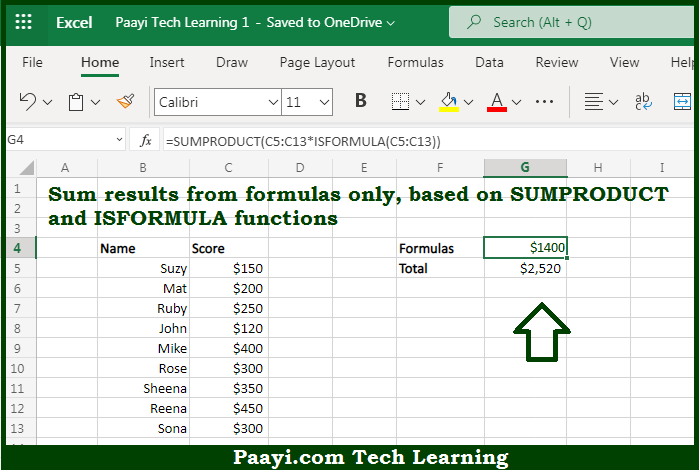# Learn How to SUM Formulas Only in Microsoft Excel

Written by | 0 Comments | 433 Views

In this article, you will learn how to SUM various things in Microsoft Excel using a single/combination(s) of functions. You will also know how to SUM Formulas Only and see the generic formula.

SUM Formulas Only in Microsoft Excel

The main purpose of this formula is to sum results from formulas only. Here we will learn how to sum formulas only given in the workbook in Microsoft Excel. That implies, with the help of a formula based on the SUMPRODUCT and ISFORMULA function you can able to sum results from formulas only. So, with the help of this formula, you can able to sum formulas only given in the workbook in Microsoft Excel.

General Formula to SUM Formulas Only

=SUMPRODUCT(range*ISFORMULA(range))

The Explanation for the SUM Formulas OnlySo we know that with the help of the given formula above you can able sum results from formulas only. Here we will learn how to sum formulas only given in the workbook in Microsoft Excel. As we know that the formula given here uses Boolean logic to "filter" the numbers in the targeted column before summing with the SUMPRODUCT function. If you evaluate the formula, values in the named range "sales" are expanded into an array. It should be noted that to filter out any values that aren't created by a formula, the ISFORMULA function is to create an array of TRUE FALSE values. So, with the help of this formula, you can able to sum formulas only given in the workbook in Microsoft Excel.